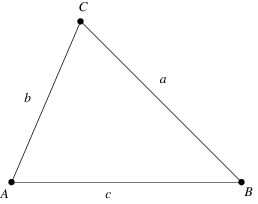## Monday, September 5, 2016

### The Triangle

An important part of testing software is making sure you have testing all cases. The other day I was talking to someone about this and they mentioned the Triangle example. I had never heard of this so he walked me through the example.A program reads three integers. The three integers are interpreted as representing the lengths of the three sides of a triangle. The program prints a message that states whether the triangle is scalene, isosceles, or equilateral. Write a set of test cases that you believe will adequately test the program.
I thought about it for a minute and was able to come up with 15 different cases to test this program. He told me that the average was 10 and that it had over 25 test cases to thoroughly test the program. I asked what all the test cases were and they are as follows:
1. You need a test case for a valid scalene triangle
2. You need a test case for a valid isosceles triangle
3. You need a test case for a valid equilateral triangle
4. You need 3 test cases for all permutations of an isosceles triangle
5. You need 3 test cases for one of the numbers being 0
6. You need 3 test cases for one of the numbers being a negative
7. You need 3 test cases where the length of one side equals the sum of the others
8. You need 3 test cases where the length of one side is greater than the sum of the others (checking to see if it is a triangle)
9. You need your null case (where all the inputs are 0’s)
10. You need 4 test cases for decimal numbers
11. You need 4 test cases of letters (specifically characters)
12. You need a test case for only 2 inputs
13. You need a test case for 4 inputs
14. For each one of the test cases you also need to figure out what the output is supposed to be

I thought this exercise was really intriguing because of the simplicity of the program and the number of test cases that needed to be checked. I normally don’t ask questions with my blogs but I would love to hear what your thoughts are on this thought exercise and what is one of your favorite testing thought exercises?

#### 5 comments :

1.This comment has been removed by the author.

1.Using BVA and EP you would reduce the number of test cases but I don't think as much as you would think. In your opinion if you were to do this using BVA and EP how many test cases would there be?

2.I think there are way too many duplicate test cases which just breeds in redundancy. Taking the using of decimals; most modern programming languages, when doing a basic cast, will strip the decimal part and just use the integer. Therefore a single test case to ensure that the application doesn't fail upon entering a non-numeric character should be sufficient. This can obviously apply to using alpha-numeric characters as well. Additionally if you are worried about the fields working differently you can use different fields for the 2 different tests.

Saying that, a completely black-box approach might require this many test cases as you cannot know how the application will react to the different scenarios.

3.I love questions like this. This would be an awesome interview question.

A question I like to ask is: Write a program (any language) that takes in an integer, and prints the integer with the correct ordinal (i.e.: 1 = 1st, 2 = 2nd, etc.). Then determine what test cases you'd need. There are some gotchas that can trip people up early in the sequence.

1.Michael,
I've seen it used as an interview question, and it is a waste of time. Why not just ask the question of how do you optimize the number of test cases for a given functionality. I do, and then if people explain how they use Boundary Value Analysis (BVA) and Equivalence Class Partitioning (EQP) then I have good insight to this persons abilities. Both BVA and EQP are basic methods in testing. Anyone who has read a decent book on Software Testing will know of these.

Asking "gotcha" questions is stupid, and insulting to the interviewee. I actually stopped an interview once because of this. I just looked at the two people asking these questions and said if you really want to know if I can do the job then ask me questions that are germane to the position.

If someone asks me the Triangle question I'll just walk on them.